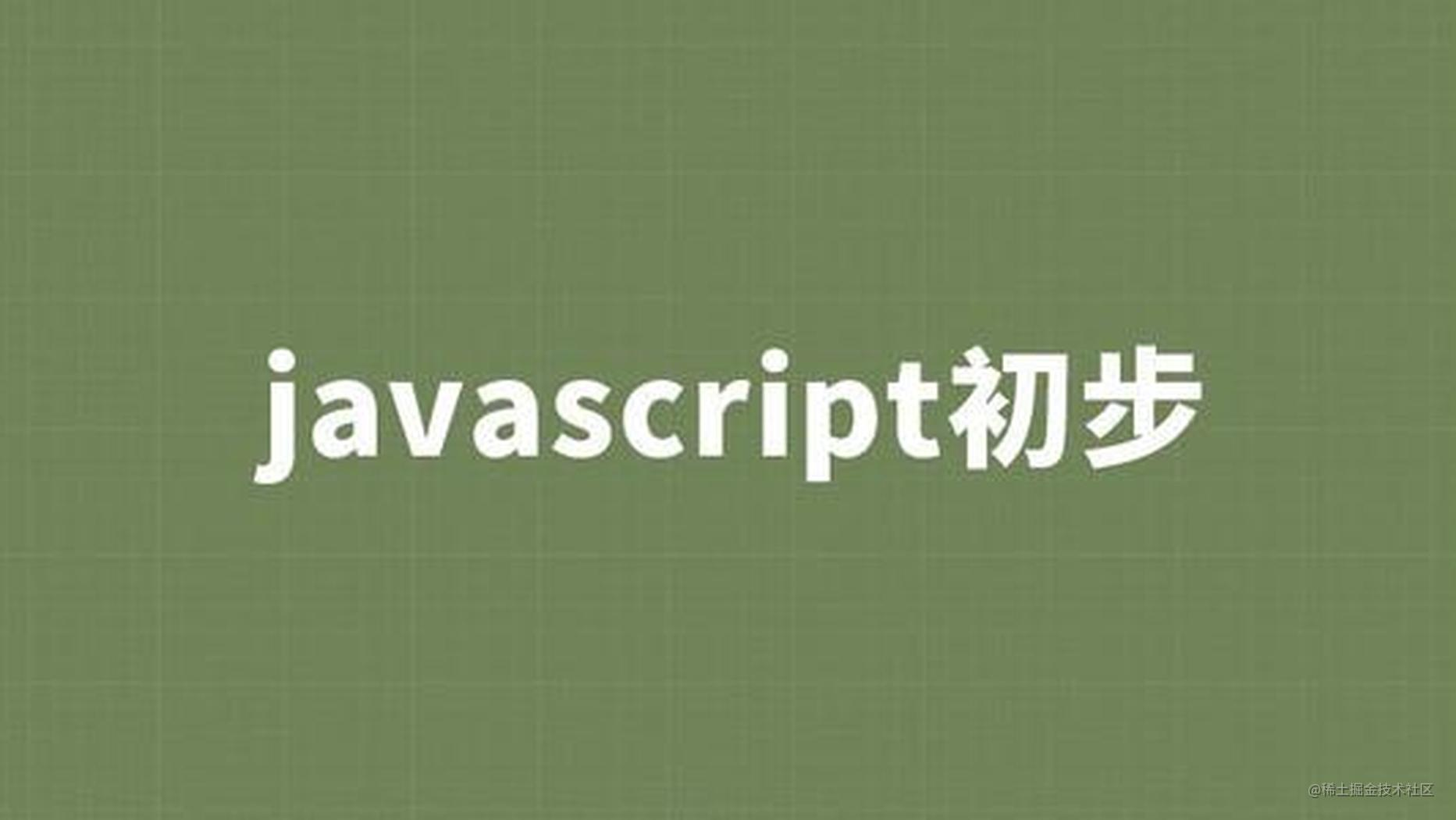# 让你快速的掌握JavaScript 数据类型## 一、JavaScript数据类型

JS中分为七种数据类型，八种内置类型又分为两大类型：7种基本类型和Object，基本类型存储在栈内存中，数据大小确定，内存空间大小可以分配，按值存放，所以可直接访问

### 1.1基本(值)类型

• Number: 任意数值
• String: 任意文本
• Boolean: true/false
• undefined: undefined
• null: null
• symbol：(ECMAScript 6 新定义)
• BigInt(ECMAScript 2020 新增)

### 1.2对象(引用)类型

• Object: 任意对象
• Array: 特别的对象类型(下标/内部数据有序)
• Function: 特别的对象类型(可执行)

## 二、数据类型判断

### 2.1通过typeOf 判断

typeof是一个操作符，其右侧跟一个一元表达式，并返回这个表达式的数据类型。返回的结果用该类型的字符串(全小写字母)形式表示，包含这8种： number、bigInt、boolean、symbol、string、object、undefined、function，

Undefined"undefined"
Null"object"
Boolean"boolean"
Number"number"
BigInt(ECMAScript 2020 新增)"bigInt"
String"string"
Function 对象 (按照 ECMA-262 规范实现 [[Call]])"function"

typeof 运算符后接操作使用方法：typeOf operand 和 typeOf()

``````typeof "";  //string
typeof 1;   //number
typeof false; //boolean
typeof undefined; //undefined
typeof function(){}; //function
typeof {}; //object
typeof Symbol(); //symbol
typeof null; //object
typeof []; //object
typeof new Date(); //object
typeof new RegExp(); //object

`由此可以看出typeof 只能判断数值, 字符串, 布尔值, undefined, function，对于null与对象, 一般对象与数组无法区分`

### 2.2通过instanceof 判断

instanceof用来判断A是否为B的实例，表达式为：`A instanceof B`，如果A是B的实例，则返回true，否则返回false。instanceof检测的是原型，内部机制是通过判断对象的原型链中是否有类型的原型。

instanceof 只能用来判断两个对象是否属于实例关系， 而不能判断一个对象实例具体属于哪种类型。

``````{} instanceof Object; //true
[] instanceof Array;  //true
[] instanceof Object; //true
"123" instanceof String; //false
new String(123) instanceof String; //true
new Date() instanceof Date;//true
new RegExp() instanceof RegExp//true
null instanceof Null//报错
undefined instanceof undefined//报错

``````function instance(left, right) {
//获取类型的原型
let prototype = right.prototype;
//获取对象的原型
let proto = left.__proto__;
//循环判断对象的原型是否等于类型的原型，直到对象原型为null，因为原型链最终为null
while (true) {
if (proto === null || proto === undefined) {
return false;
}
if (proto === prototype) {
return true;
}
proto = proto.__proto__;
}
}

``````// 为了方便表述，首先区分左侧表达式和右侧表达式
ObjectL = Object, ObjectR = Object;
// 下面根据规范逐步推演
O = ObjectR.prototype = Object.prototype
L = ObjectL.__proto__ = Object.prototype
// 第一次判断
O != L
// 循环查找 L 是否还有 __proto__
L = Function.prototype.__proto__ = Object.prototype
// 第二次判断
O == L
// 返回 true

`综上所述：instanceof专门用来判断对象数据的类型: Object, Array与Function`

### 2.3 通过constructor 判断

`constructor是原型prototype的一个属性`，当函数被定义时候，js引擎会为函数添加原型prototype，并且这个prototype中constructor属性指向函数引用， 因此重写prototype会丢失原来的constructor。 从原型链角度讲，构造函数就是新对象的类型。这样做的意义是，让对象诞生以后，就具有可追溯的数据类型

``````(2).constructor === Number // true
'string'.constructor === String // true
(true).constructor === Boolean // true
([]).constructor === Array // true
(function() {}).constructor === Function // true
({}).constructor === Object // true
new Date().constructor === Date // true

``````function Fn(){}
Fn.prototype = []

const fn = new Fn()
f.constructor=== Fn // false
f.constructor=== Array // true

`需要注意：null 和 undefined 无constructor，将无法通过无constructor判断类型`

### 2.4 通过 toString() 判断

toString()是Object的原型方法，调用该方法，默认返回当前对象的[[Class]]。这是一个内部属性，其格式为[object Xxx],其中Xxx就是对象的类型。

``````// 1. 判断基本类型
Object.prototype.toString.call(null); // "[object Null]"
Object.prototype.toString.call(undefined); // "[object Undefined]"
Object.prototype.toString.call(“abc”);// "[object String]"
Object.prototype.toString.call(123);// "[object Number]"
Object.prototype.toString.call(true);// "[object Boolean]"

// 3.引用类型
Object.prototype.toString.call(function fn(){}); // "[object Function]"
Object.prototype.toString.call({}); // "[object Object]"
Object.prototype.toString.call(new Date()); // "[object Date]"
Object.prototype.toString.call([]); // "[object Array]"

``````console.log("jerry".toString()) // jerry
console.log((1).toString()) // 1
console.log([1,2].toString()) // 1,2
console.log(new Date().toString()) // Wed Dec 21 2016 20:35:48 GMT+0800 (中国标准时间)
console.log(function(){}.toString()) // function (){}
console.log(null.toString()) // error
console.log(undefined.toString()) // error

## 三、类型转换

### 3.1 转换为String类型

``````// 将Nubber ---> String
let a = 100
let b = a.toString() // '100'
console.log(a) // 100

// 将Boolean --> String
let c = true
let d = c.toString() // "true"

``````// 将Nubber ---> String
let a = 100
let b = String(a) // '100'
console.log(a) // 100

// 将Boolean --> String
let c = true
let d = String(c) // "true"

// null --> String
let e = null
let f = String(e) // "null"

// undefined --> String
let g = String(undefined)
let h = String(e) // "undefined"

`注意：当你在使用toString时,需要判断被转换的值不能为null 和 undefined`

### 3.2 转换为Number类型

• 可以字符串 --> Number

• 1.如果是纯数字的字符串，则直接将其转换为数字；
• 2.如果字符串中有非数字的内容，则转换为NaN；
• 3.如果字符串是一个空串或者是一个全是空格的字符串，则转换为0
• 布尔 --> Number

• null --> Number

• undefined --> Number

``````// 纯数字字符串 --> Number
let a = '100'
Number(a) // 100

// 非数字字符串 --> Number
let a = 'aaaa'
Number(a) // NaN

// 空串或者是一个全是空格的字符串
let a = '     '
Number(a) // 0

// 布尔 --> Number
Number(true) // 1
Number(false) // 0

// null --> Number
Number(null) // 0

// undefined --> Number
Number(undefined) // NaN

` parseInt(string, radix) 解析一个字符串并返回指定基数的十进制整数， radix 是2-36之间的整数，表示被解析字符串的基数，然后返回一个整数或 NaN。`

• string要被解析的值。如果参数不是一个字符串，则将其转换为字符串(使用 ToString 抽象操作)。字符串开头的空白符将会被忽略。

• radix 可选, 2 到 36，表示字符串的基数。例如指定 16 表示被解析值是十六进制数。请注意，10不是默认值！，`如果 36 < radix < 2 时 parseInt 结果将会返回NaN`

• 再利用parseInt时，string要被解析的值包含了非数字字符时,parseInt 将会截取非数字字符串部分之前的字符返回

• 如果对非String使用parseInt()，它会先将其转换为String然后在操作

``````// 示范
parseInt('123', 5) // 将'123'看作5进制数，返回十进制数38 => 1*5^2 + 2*5^1 + 3*5^0 = 38

// 将字符串'1111' ---> Number
parseInt('111') // 111

// 非String ---> Number
parseInt('111px222') // 111
parseInt('100.22') // 100

// 容易出错的使用
parseInt(null) // NaN
parseInt(undefined) //NaN
parseInt() // NaN
parseInt('') // NaN
parseInt('a1212') // NaN
parseInt('1111', 1) // NaN

``````// 接下来对这个题展开处理，1.考察数组map的方式使用，2.对parseInt 的使用
var new_array = [].map(function callback(currentValue[, index[, array]]) {
// Return element for new_array
}[, thisArg])

// currentValue : 当前的项
// index: 当前的索引

// 所以上面的代码转化为：
["1", "2", "3"].map((item, index) => parseInt(item, index))

// 经过上一步会得到
[parseInt("1", 0),parseInt("2", 1),parseInt("3", 2)]

// 因为parseInt 的 radix 参数 取值范围值 2 到 36
[1, NaN, NaN]

### 3.3 转换为Boolean

• 数字 ---> 布尔，除了0和NaN，其余的都是true
• 字符串 ---> 布尔，除了空串，其余的都是true
• null和undefined都会转换为false
• 对象也会转换为true
``````// 数字 ---> 布尔
Boolean(1) // true
Boolean(0) // false
Boolean(-100) // true
Boolean(Infinity) // true
Boolean(NaN) // false

// 字符串 ---> 布尔
Boolean('') // false
Boolean('aaa') // true

//  null和undefined --->布尔
Boolean(null) // false
Boolean(undefined) // false

// 对象 ---> 布尔
Boolean({}) // true
Boolean([]) // true
Boolean(Function) // true

## 四、基本运算

`当对非Number类型的值进行运算时，会将这些值转换为Number然后在运算(字符串除外)，任何值和NaN做运算都得NaN， 任何值做- * /运算时都会自动转换为Number`

``````// 加法
console.log(1 + '') // '1'
console.log(1 + 'a') // '1a'
console.log('a' + 'b') // 'ab'
console.log(true + false) // ==>console.log(1 + 0)
console.log(1 + null) // ==> 1+ Number(null) ==> 1+0
console.log(1 + undefined) // 1+ Number(undefined) ==> 1+NaN=NaN
console.log(100 + []) // 100 + [].toString() ==> '100'

`任何值和字符串相加都会转换为字符串，并做拼串操作，我们可以利用这一特点，来将一个任意的数据类型转换为String，这是一种隐式的类型转换，由浏览器自动完成，实际上它也是调用String()函数`

``````console.log('100' - 0) // Number('100') - 0 = 100
console.log('100' * 2) // Number('100') * 2 = 200
console.log('100' / 2) // Number('100') / 2 = 50

``````let result = 100 + true + 21.2 + null + undefined + "Tencent" + [] + null + 9 + false

// 步骤1
100 + true = 100 + 1 = 101 + 21.2 = 122.2 + Number(null) = 122.2

// 步骤2
122.2 + Number(undefined) = 122.2 + NaN = NaN

// 步骤3
NaN + "Tencent" =  "NaNTencent"

// 步骤4
"NaNTencent" + [] = "NaNTencent" + [].toString() = "NaNTencent" + '' = "NaNTencent"

// 步骤5
"NaNTencent" + null = "NaNTencent"  + null.toString() = "NaNTencentnull"

// 步骤6
"NaNTencentnull" + false = "NaNTencentnull" + false.toString() = "NaNTencentnullfalse"

// 最终的结果： NaNTencentnullfalse